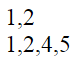Support us .Net Basics C# SQL ASP.NET Aarvi MVC Slides C# Programs Subscribe Download

### Difference between each and map in jquery

Suggested Videos
Part 25 - jquery add or remove class
Part 26 - Difference between \$.each and .each
Part 27 - jQuery map method

In this video we will discuss the difference between each and map functions in jquery

 \$.map \$.each map method can be used as an iterator. each method is an immutable iterator Returns a new array Returns the original array The order of callback arguments - element, index. \$.map(elems, function () { element, index }, arg) The order of callback arguments - index, element. \$.each(elems, function () { index, element }, arg) Does not have a way to terminate the iteration Return false to terminate the iteration

Example : Notice that the callback arguments in the each method are the reverse of the callback arguments in the map function. Also notice that map returns a new array where as each method returns the original array. This proves the point that each method is an immutable iterator where as map is not.

var intArray = [1, 2, 3, 4, 5];

function functionA(index, element) {
return element * 5;
}

function functionB(element, index) {
return element * 5;
}

var result1 = \$.each(intArray, functionA);
var result2 = \$.map(intArray, functionB);

document.write('each = ' + result1);
document.write('<br/>')
document.write('map = ' + result2);
});

Output :Example : Notice that each method terminates the iteration when the element value is 3. The values 3, 4 and 5 are not written to the document. With map method we are not able to break the iteration. When the element value is 3, map method returns false and then continues writing 4 and 5 to the document.

var intArray = [1, 2, 3, 4, 5];

\$.each(intArray, function (index, element) {
if (element == 3)
return false;

document.write(element + ',');
});

document.write('<br/>');

\$.map(intArray, function (element, index) {
if (element == 3)
return false;

document.write(element + ',');
});
});

Output :